# Fermat prime greater than three implies three is primitive root

## Statement

Supposeis a positive integer (in particular, it is not zero) such that the Fermat numberis a prime number (and hence a Fermat prime). Then, the number 3 is a quadratic nonresidue modulo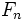, and hence a primitive root modulo.

## Proof

We use Fact (1), which says that, in terms of Legendre symbols: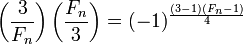Note that:

• Since,, so the exponent on the right side is even, so the right side is 1.
• We know that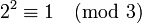, so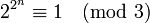, so. Thus,which is -1.

Plugging these in, we get: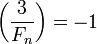Thus, 3 is a quadratic nonresidue mod. Combine with Fact (2) to obtain that it is a primitive root.